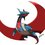# Proof of combo formula using analytical NT

claim: $\sum_{a=0}^n \binom{a+k}{k}=\binom{n+k+1}{k+1}$ (wierd) proof: Consider Induction on: $f_k(p^a)=(\underbrace{1*1*1*1*1\cdots}_{k\text{ times}})(p^n)=\binom{k+n}{k}$ then $f_{k+1}(p^a)=(\underbrace{1*1*1*1*1\cdots}_{k\text{ times}}*1)(p^n)=\sum_{p^a|p^n}\binom{k+a}{k}=\sum_{a=0}^n \binom{k+a}{k}$ If our original claim is true, then $(\underbrace{1*1*1*1*1\cdots}_{k\text{ times}}*1)(p^n)=\binom{k+n+1}{k+1}$ Now i am going to use dirichlet series to prove this to be true: $\zeta^k(s)=\sum_{i=1}^\infty \dfrac{f_k(i)}{i^s}\\=\prod_{p}\sum_{a=0}^\infty \dfrac{\binom{k+a}{k}}{p^{as}}=\prod_{p}\dfrac{1}{k!}\sum_{a=0}^\infty \dfrac{(a+1)(a+2)...(a+k)}{(p^{s})^a}$ By continuously differentiating a version of GP(exercise for the reader), we have $\sum_{a=0}^\infty (a+1)(a+2)...(a+k)x^a=\dfrac{k!}{(1-x)^k}$ Putting this in the product: $\zeta^k(s)=\prod_p \dfrac{1}{(1-p^{-s})^k}=\zeta^k(s)$ $s=s$ Hence proved.Note by Aareyan Manzoor
4 years, 11 months ago

This discussion board is a place to discuss our Daily Challenges and the math and science related to those challenges. Explanations are more than just a solution — they should explain the steps and thinking strategies that you used to obtain the solution. Comments should further the discussion of math and science.

When posting on Brilliant:

• Use the emojis to react to an explanation, whether you're congratulating a job well done , or just really confused .
• Ask specific questions about the challenge or the steps in somebody's explanation. Well-posed questions can add a lot to the discussion, but posting "I don't understand!" doesn't help anyone.
• Try to contribute something new to the discussion, whether it is an extension, generalization or other idea related to the challenge.

MarkdownAppears as
*italics* or _italics_ italics
**bold** or __bold__ bold
- bulleted- list
• bulleted
• list
1. numbered2. list
1. numbered
2. list
Note: you must add a full line of space before and after lists for them to show up correctly
paragraph 1paragraph 2

paragraph 1

paragraph 2

[example link](https://brilliant.org)example link
> This is a quote
This is a quote
    # I indented these lines
# 4 spaces, and now they show
# up as a code block.

print "hello world"
# I indented these lines
# 4 spaces, and now they show
# up as a code block.

print "hello world"
MathAppears as
Remember to wrap math in $$ ... $$ or $ ... $ to ensure proper formatting.
2 \times 3 $2 \times 3$
2^{34} $2^{34}$
a_{i-1} $a_{i-1}$
\frac{2}{3} $\frac{2}{3}$
\sqrt{2} $\sqrt{2}$
\sum_{i=1}^3 $\sum_{i=1}^3$
\sin \theta $\sin \theta$
\boxed{123} $\boxed{123}$

Sort by:

Looks like your claim is a corollary of this. By the way, the proof was interesting :).

- 4 years, 11 months ago

never seen that before

- 4 years, 11 months ago

I came across it while spending time with simple binomial sums, and thought that would make a good problem on brilliant.

- 4 years, 11 months ago

Actually, I initially derived $1*1*...*1 \text{ Convoluted k times }(p^n) = \binom{k+n}{k}$ by proving via induction that $1*1*...*1 \text{ Convoluted k times }(p^n)=\sum_{0 \le a_1 \le a_2 ... \le a_{k} \le n}1$ and thereafter using the solution in this beautiful problem to evaluate the final answer.

So another alternative to proving the above identity is to just use that problem

- 4 years, 11 months ago

Use \substack ;)

- 4 years, 11 months ago

Initially i derivd $1*1*1*1....\text{k times}(p^a)=\dfrac{(a+1)(a+2)(a+3)...(a+k)}{k!}$ which i turned into binomial coefficient later.

There are obviously easier proof.... This was intended as a more interesting/wierd proof.

- 4 years, 11 months ago

Feel free to fill out the "exercise for reader" in the comment.

- 4 years, 11 months ago